# Truncated trihexagonal tiling

Truncated trihexagonal tiling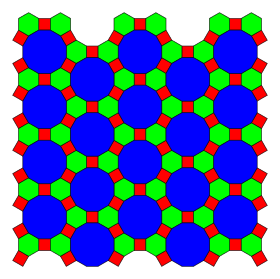TypeSemiregular tiling
Vertex configuration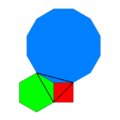4.6.12
Schläfli symboltr{6,3} orWythoff symbol2 6 3 |
Coxeter diagramSymmetryp6m, [6,3], (*632)
Rotation symmetryp6, [6,3]+, (632)
Bowers acronymOthat
DualKisrhombille tiling
PropertiesVertex-transitive

In geometry, the truncated trihexagonal tiling is one of eight semiregular tilings of the Euclidean plane. There are one square, one hexagon, and one dodecagon on each vertex. It has Schläfli symbol of tr{3,6}.

## Other names

• Great rhombitrihexagonal tiling
• Rhombitruncated trihexagonal tiling
• Omnitruncated hexagonal tiling, omnitruncated triangular tiling
• Conway calls it a truncated hexadeltille, constructed as a truncation operation applied to a trihexagonal tiling (hexadeltille).

## Uniform colorings

There is only one uniform coloring of a truncated trihexagonal tiling, with faces colored by polygon sides. A 2-uniform coloring has two colors of hexagons. 3-uniform colorings can have 3 colors of dodecagons or 3 colors of squares.

1-uniform 2-uniform 3-uniform
Coloring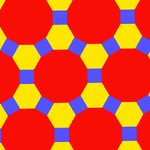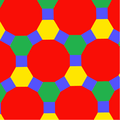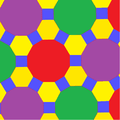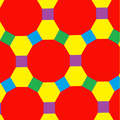Symmetry p6m, [6,3], (*632) p3m1, [3], (*333)

The truncated trihexagonal tiling has three related 2-uniform tilings, one being a 2-uniform coloring of the semiregular rhombitrihexagonal tiling. The first dissects the hexagons into 6 triangles. The other two dissect the dodecagons into a central hexagon and surrounding triangles and square, in two different orientations.

Semiregular Dissected 2-uniform 3-uniform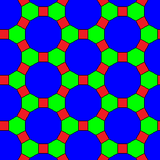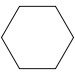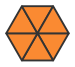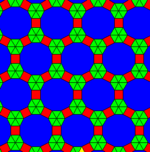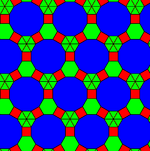Dissected Semiregular 2-uniform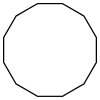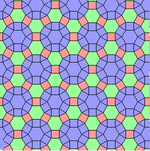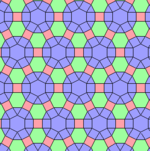## Circle packing

The Truncated trihexagonal tiling can be used as a circle packing, placing equal diameter circles at the center of every point. Every circle is in contact with 3 other circles in the packing (kissing number). Circles can be alternatedly colored in this packing with an even number of sides of all the regular polygons of this tiling.

The gap inside each hexagon allows for one circle, and each dodecagon allows for 7 circles, creating a dense 4-uniform packing.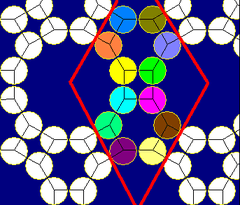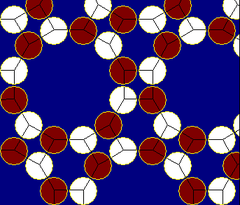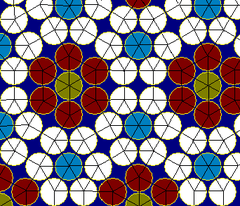## Kisrhombille tiling

Kisrhombille tiling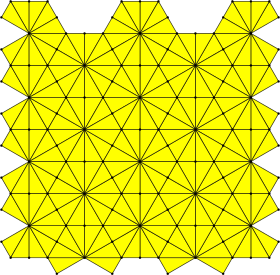TypeDual semiregular tiling
Coxeter diagramFaces30-60-90 triangle
Face configurationV4.6.12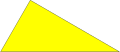Symmetry groupp6m, [6,3], (*632)
Rotation groupp6, [6,3]+, (632)
Dualtruncated trihexagonal tiling
Propertiesface-transitive

The kisrhombille tiling or 3-6 kisrhombille tiling is a tiling of the Euclidean plane. It is constructed by congruent 30-60 degree right triangles with 4, 6, and 12 triangles meeting at each vertex.

### Construction from rhombille tiling

Conway calls it a kisrhombille for his kis vertex bisector operation applied to the rhombille tiling. More specifically it can be called a 3-6 kisrhombille, to distinguish it from other similar hyperbolic tilings, like 3-7 kisrhombille.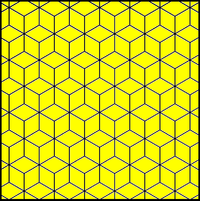The related rhombille tiling becomes the kisrhombille by subdividing the rhombic faces on it axes into four triangle faces

It can be seen as an equilateral hexagonal tiling with each hexagon divided into 12 triangles from the center point. (Alternately it can be seen as a bisected triangular tiling divided into 6 triangles, or as an infinite arrangement of lines in six parallel families.)

It is labeled V4.6.12 because each right triangle face has three types of vertices: one with 4 triangles, one with 6 triangles, and one with 12 triangles.

### Practical uses

The kisrhombille tiling is a useful starting point for making paper models of deltahedra, as each of the equilateral triangles can serve as faces, the edges of which adjoin isosceles triangles that can serve as tabs for gluing the model together.

## Symmetry

The kisrhombille tiling triangles represent the fundamental domains of p6m, [6,3] (*632 orbifold notation) symmetry. There are a number of small index subgroups constructed from [6,3] by mirror removal and alternation. [1+,6,3] creates *333 symmetry, shown as red mirror lines. [6,3+] creates 3*3 symmetry. [6,3]+ is the rotational subgroup. The communtator subgroup is [1+,6,3+], which is 333 symmetry. A larger index 6 subgroup constructed as [6,3*], also becomes (*333), shown in blue mirror lines, and which has its own 333 rotational symmetry, index 12.

There are eight uniform tilings that can be based from the regular hexagonal tiling (or the dual triangular tiling). Drawing the tiles colored as red on the original faces, yellow at the original vertices, and blue along the original edges, there are 8 forms, 7 which are topologically distinct. (The truncated triangular tiling is topologically identical to the hexagonal tiling.)

### Symmetry mutations

This tiling can be considered a member of a sequence of uniform patterns with vertex figure (4.6.2p) and Coxeter-Dynkin diagram. For p < 6, the members of the sequence are omnitruncated polyhedra (zonohedra), shown below as spherical tilings. For p > 6, they are tilings of the hyperbolic plane, starting with the truncated triheptagonal tiling.Wikimedia Commons has media related to Uniform tiling 4-6-12.